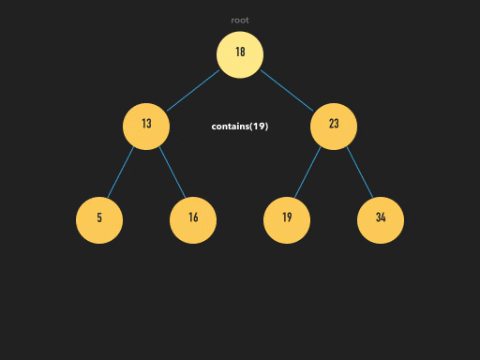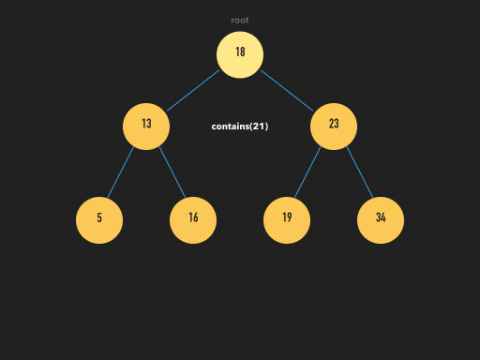## DEV Community 👩‍💻👨‍💻

Jenny Shaw

Posted on • Updated on

# Binary Trees (Part 2) - Binary-Search Trees are the BeST

In this blog, I'll be covering Binary-Search Trees, focusing primarily on BST structuring, how to create a BST class, insert new nodes, and check for a value in Javascript.

## What are Binary-Search Trees?

Binary-Search Trees (BSTs) are a binary tree data structure that come with a special quality -- sortedness.

A BST is naturally sorted, which makes searching for a value extremely efficient and quick. And the BST class possesses methods to insert and delete nodes in ways that always preserve and maintain that sorted state.

Nodes in a binary tree can point to no more than two children. In a BST, however, there are additional supreme rules about a node's location in relation to other nodes, and this is to maintain the hierarchical order of the tree.

Each parent node points to a left child and/or a right child. If a child's value is less than the parent's, the child must be the left child node. On the other hand, if the child's value is greater, then that child must be the right child node.## Code Break: Node and BST Classes

Let's build out the basic pieces of a BST in Javascript.

First, we'd write out a Node class. A node would have a value property which contains the value used as we initialize a node object. It would also have references to a left node and a right node, both of which will be null since at the moment of its creation it'll just be a standalone node.

``````class Node {
constructor(value) {
this.value = value;
this.left = null;
this.right = null;
}
}
``````

To start building out the tree, we would also create a BST class. The class would contain a reference to the root, and because a new tree begins with a new node, the root would be the first newly initialized node.

``````class BST {
constructor(value) {
this.root = new Node(value);
this.count = 1;
}
}
``````

You might've noticed that I also added another property to BST called `count`. It refers to the number of nodes existing in the tree, and it'll be useful when you want to keep track of your node count as you're inserting or deleting nodes.

## BST Method: Node Insertion

So, in the event that we want to insert a new node into a tree, we have to consider its value. A new node's value determines our path through the tree's branches all the way to the very end. It a potentially zigzagging journey all the way to the bottom.

At every node that we visit, the new node compares its own value with the currently visited node to determine whether we should follow the left or right path from there. If the new node's value is smaller, we'll travel further left, or if it's larger, then we'll travel further right.

And finally, when we reach a node where the next direction we'd want to follow points to null, we then point the current node to our new node and complete the insertion.## Code Break: Insert Method

Inside of the BST class, following the constructor, we'll create a method called `insertNode` which will do what we just described above.

First we'll initialize the new Node that we want to insert.

``````// insert method inside of BST class
insertNode(value) {
let newNode = new Node(value);
this.count++;
}
``````

Then, we need a helper method, `search`, to help us with two tasks.

The first is to search for the appropriate path from the current node to the next -- in other words, it chooses whether we go left or right.

The second is to determine if there's a node following that path. If there isn't, the `search` inserts the new node by pointing the current node to it. However, if there is, we'd continue in that direction and visit the next node where we start the search cycle all over again.

This search cycle can be accomplished recursively.

``````// write search helper method inside of insertNode() method

const search = node => {
//if the new node value is less than the current node value, we'll look left
if (value < node.value) {
// if there's no left child,
if (!node.left) {
// then insert the new node
node.left = newNode;
} else {
// search the left node by calling the method on it
// (yay, recursion!)
search(node.left);
}
// if new node is greater than current node, we'll look right
// repeat similar logic
} else {
if (!node.right) {
node.right = new Node;
} else {
search(node.right)
}
}
}
``````

To wrap the `insertNode` method up, we'd call `search` on the root. This starts the search beginning on the root and then on every node that we visit thereafter.

``````// at the end of insertNode method...

search(this.root);
``````

Here's the entire method in a single snippet.

``````insertNode(value) {
let newNode = new Node(value);
this.count++;

const search = node => {
if (value < node.value) {
if (!node.left) {
node.left = newNode;
} else {
search(node.left);
}
} else {
if (!node.right) {
node.right = new Node;
} else {
search(node.right)
}
}
}
search(this.root);
}
``````

## BST Method: Checking if a Tree Contains a Value

Now let's see if we can find target values!

If I were to search for a value in a BST, it'd super quick. Even in your worst-case scenario, it wouldn't even have a time complexity of O(N) (meaning that you had visited and processed every single node on the tree) but of O(log N). You would never have to process more than half of the values in a tree to find your target.

Remember when I mentioned that the left child always has a smaller value than the parent, meanwhile the right child has a greater value? Because it's set up this way, every time I compare the value I'm searching for with a node and as soon as I've decided whether to visit the left or right subtree, I've essentially discarded the other half of the tree. And each time I do this on a new node, I'm discarding my remaining search pile by half, thus saving significant time and effort.

Below is an example of a successful search for the target value on a tree.And below here is how we search and conclude that the target value doesn't exist.## Code Break: Contains Method

First, we start our search from the top of the tree. We'll want to establish a current node, a marker to help us keep track of our location on the tree as we travel down it. We'll start the marker at the root by assigning `this.root` to `current`.

Then we'll do two things. First, we'll compare the target value to the current node value and see if they match. If they do, we return true, and we're done! If they don't match, then we'll do the second thing, move down the tree one node. If the target value is less than the current value, then we'll move on to the left node by assigning the left node to `current`. Otherwise, the right node is `current`. When the loop is complete, we'll repeat the process on the following node. If we've searched the tree from top to bottom with no success, then we break out of the loop and simply return false.

``````// add a new method to BST class

contains(value) {
let current = this.root;

while(current !== null) { // while there is a current node
// compare values

// is it a match?
if (value === current.value) {
return true;

// if not, move down a node
} else if (value < current.value) {
current = current.left;
} else {
current = current.right;
}
}
return false;
}
``````

## Conclusion

Binary-Search Trees are one of the most satisfyingly useful and efficient data structures. Once you understand the structure, they're rather intuitive and easy to understand. And because they're already sorted, they're excellent for searches, insertions, and deletions. Deletions are a little more complicated than the methods I covered here, so I'll be writing more about it in the next blog. Stay tuned!

For more information on binary trees, check out these other blogs from my 5-part binary tree series!